### Finding Roots of Equations: Problems

1. Let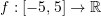be such that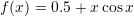. Use the graphical method to find the number and estimates of the roots of each of the equations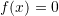and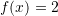.
2. Compare the bisection method and the false position method in finding the roots of the two equationsand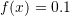in the interval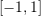where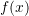is: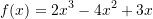Compare with the result using the Solve function and comment on the use of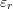as a measure of the relative error.
3. Locate the first nontrivial root of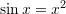whereis in radians. Use the graphical method and the bisection method with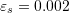.
4. Water is flowing in a trapezoidal channel at a rate of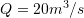. The critical depth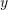for such a channel must satisfy the equation: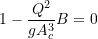where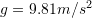,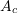is the cross sectional area (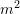), and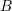is the width of the channel at the surface (). Assuming that the width and the cross‐sectional area can be related to the depthby: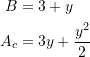Solve for the critical depth using the graphical method, the bisection method, and the false position method to find the critical depth in the interval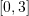. Use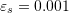. Discuss your results (difference in the methods, accuracy, number of iterations, etc.).

(Note: The critical depth is the depth below which the flow of the fluid becomes relatively fast and affected by the upstream conditions (supercritical flow). Above that depth, the flow is subcritical, relatively slow and is controlled by the downstream conditions)

5. Use the graphical method to find all real roots of the equation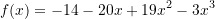You are required to

• Plot the function several times by using smaller ranges for theaxis to obtain the roots up to 3 significant digits.
• Explain the steps you have taken to identify the number and estimates of the roots.
• Explain the steps you have taken to obtain the roots to the required accuracy.
• Compare your results to the solution obtained using the “Solve” function in Mathematica.
6. Use the bisection method and the false position method to find all real roots of the equationUse.
7. Consider the function: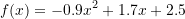Determine a root for the expression• using fixed-point iteration
• using the Newton-Raphson method

Use an initial guess of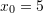and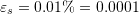. Compare your final answer with the Solve function in Mathematica.

8. Consider the function: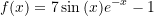Find the lowest positive root for the expression• graphically
• using the Newton-Raphson method
• using the secant method
9. Use the Newton-Raphson method to find the root of: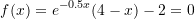Employ initial guesses of 2, 6, and 8. Explain your results.
10. The Manning equation can be written for a rectangular open channel as: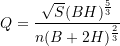where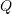is the fluid flow in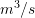,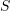is the slope in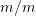,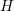is the depth in, andis the Manning roughness coefficient. Develop a fixed-point iteration scheme to solve this equation forgiven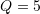,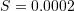,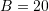, and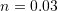. Consider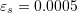. Prove that your scheme converges for all initial guesses greater than or equal to zero.
11. Why does the Babylonian method almost always converge?
12. Consider the function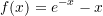Compare the secant method and the Newton-Raphson method in finding the root of the equation.
13. Solve the previous question using the Fixed-Point Iteration method
14. Use the Fixed-Point Iteration method to find the roots of the following functions: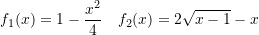15. Engineers of many disciplines use thermodynamics extensively in their work. The following polynomial can be used to relate the zero-pressure specific heat of dry air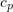kJ/(kg K) to temperature (K):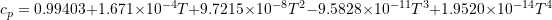Plotversus T in the range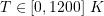​. Determine the temperature that corresponds to a specific heat of 1.1 kJ/(kg K) using the following methods. In each case use an approximate relative error tolerance of 0.0001% and report the number of iterations required to achieve that tolerance.
– Bisection Method. Set up the initial brackets [0,1200] K.
– Fixed-Point Iteration. Using an initial guess of T = 500 K.
– Newton-Raphson Method. Using an initial guess of T = 500 K.
– Secant Method. Use initial guesses of T = 540 K and T = 550K.
Make a conclusion about the efficiency of the methods.